# Reconstruction of the corridor

Calculate how many minutes will be reduced to travel 187 km long railway corridor, where the maximum speed increases from 120 km/h to 160 km/h.

Calculate how many minutes will shorten travel time, if we consider that the train must stop at 6 stations, each station with the equally slow 0.8 m/s2 and from station accelerates 0.5 m/s2?

What is the average speed before and after reconstruction, if the train stays for 2 minutes in every station?

Consider the intermediate stops are so long that the train will always reach the speed limit.

Correct result:

t1 =  23.375 min
t2 =  20.9444 min
v1 =  0 km/h
v2 =  0 km/h

#### Solution: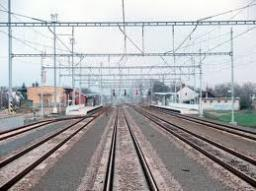We would be pleased if you find an error in the word problem, spelling mistakes, or inaccuracies and send it to us. Thank you!Tips to related online calculators
Do you have a linear equation or system of equations and looking for its solution? Or do you have quadratic equation?
Do you want to convert length units?
Do you want to convert velocity (speed) units?
Do you want to convert time units like minutes to seconds?

## Next similar math problems:

• ICE train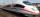German runways test a new ICE train between Munich and Berlin. The train runs to Berlin at a slow speed of 100 km/h. Back from Berlin goes faster. How quickly did the train have to go on a return trip so that the total average train speed for both journey
• Train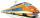The train is running at speeds of 96 km/h. From the beginning of braking to full stop train run for 3.3 minutes. If the train slows the braking equally, calculate the distance of the place from the station where you need to start to brake.
• AccelerationThe car accelerates at rate 0.5m/s2. How long travels 400 meters and what will be its speed?
• Motion 2At 10 o'clock started from place A car speed at 90 km/h. 20 minutes after started from the place B at speed 60 km/h another car. Distance places A and B is 120 kilometers. What time and where they meet?
• Two citiesCities A and B are 200 km away. At 7 o'clock from city A, the car started at an average speed of 80 km/h, and from B at 45 min later the motorcycle is started at an average speed of 120 km/h. How long will they meet and at what distance from the point A i
• Double-track line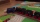A 160 m long passenger train runs on a double-track line in one direction at a constant speed of 54 km/h, and a 240 m long express train in the opposite direction. a) How fast is the express train if passing the passenger train driver for 6 s? b) How long
• Constant motionThe car has undergone constant motion 120 km in 1 hour 30 minutes. Determine its average speed. Determine how long car drove in the first 20 minutes and 50 minutes from the start of the move.
• Pedestrian and the cyclist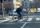Pedestrian and cyclist went against each from the two cities that are distant 40 km. Pedestrian started 30 minutes before the cyclist and walk an average speed of 5 km/h. What speed went cyclist when met after 120 minutes from the start of the pedestrian?
• Two vans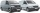At 8:30 a. M. , two vans leave at a parking area. They travel in opposite directions: one van travels at 48 km/h and the other at 62 km/h. At what time will the cars be 550 km apart?
• Average speedWhen the bus stops at bus stops driving average speed is 45 km/h. If it did not stop it drive at speed 54 km/h. How many minutes of every hour it spend at stops?
• Two trains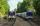Two trains go at each other. First at speed 40 km/h and the second at speed 60 km/h. At what distance will be 30 minutes before they will meet?
• Train speed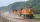The train speed is decreased during 50 sec from 72 km/h to 36 km/h. Assuming that the train movement is equally slowing, find the the acceleration and the distance that it travels at.
• Cars motion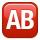From point A car start at the speed of 90 km/h. Against it departs from the point B at the same time another car at 60 km/h. For how long and where they met if the distance between A and B is 180 km?
• Two trains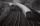There are two trains running the same distance. 1st train will travel it in 7 hours 21minutes. 2nd the train will travel 5 hours 57minutes and 34 seconds and it is 14 km/h faster than the first train. What are speeds of trains and how long is this railway
• Hurry - rush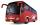At an average speed 7 km/h I will come from the school to the bus stop for 30 minutes. How fast I need to go if I need to get to the bus stop in 21 minutes?
• Two trainsThrough the bridge, long l = 240m, the train passes through the constant speed at time t1 = 21s. A train running along the traffic lights at the edge of the bridge passes the same speed at t2 = 9s. a) What speed v did the train go? b) How long did it take
• Two trains meetFrom A started at 7:15 express train at speed 85 km/h to B. From B started passenger train at 8:30 in the direction to A and at speed 55 km/h. The distance A and B are 386 1/4 km. At what time and at what distance from B the two trains meet?